### Home > INT2 > Chapter 12 > Lesson 12.2.1 > Problem12-60

12-60.
1. Perry throws a tennis ball up into the air from the edge of a cliff. The height of the ball can be modeled by the function y = −16x2 + 64x + 80, where y represents the height in feet of the ball above ground at the bottom of the cliff, and x represents the time in seconds after the ball is thrown. Homework Help ✎

1. How high is the ball when it is thrown? How do you know?

2. What is the height of the ball three seconds after it is thrown? What is its height a half second after it is thrown? Show all work.

3. When does the ball hit the ground? Write and solve an equation that represents this situation.

4. What is the domain of the function for this context?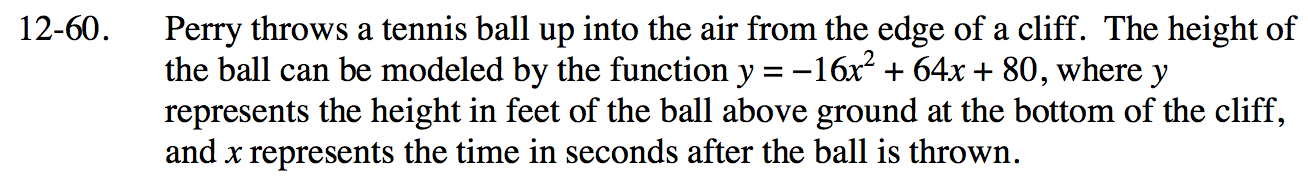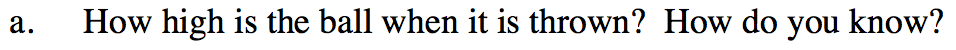If you sketch the curve, it is a downward-facing parabola. Where is the highest point?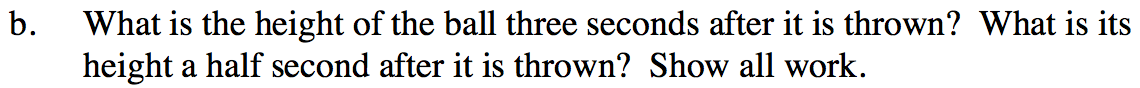y = −16(3)2 + 64(3) + 80
y = −16(0.5)2 + 64(0.5) + 80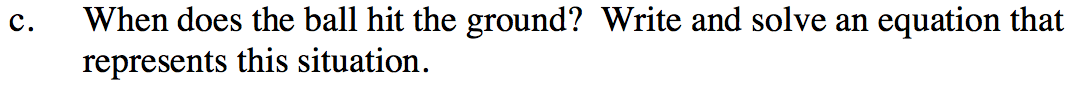The height of the ball when it hits the ground is 0 feet.

Solve 0 = −16(x)2 + 64(x) + 80.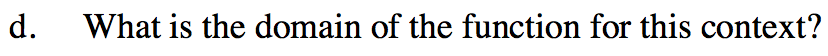This is the time from when the ball is initially thrown until the time it hits the ground.